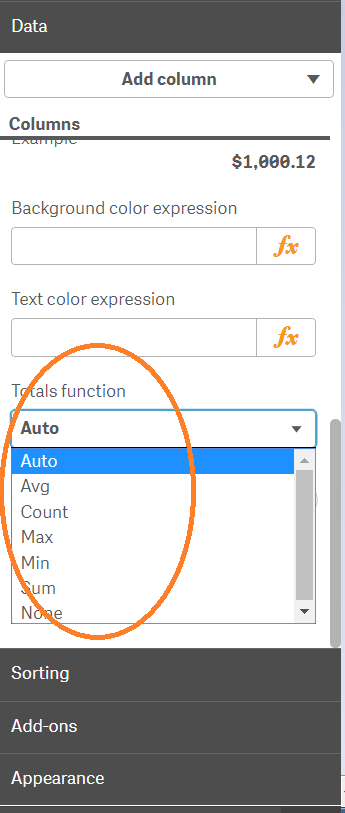# New to Qlik Sense

Discussion board where members can get started with Qlik Sense.

Highlighted
Contributor

## Totals function straight table

What does the 'Auto' aggregation perform for the totals function? It's not average/mean; I tried that and the total was different. I tried the other total functions ie max, min, count etc. and 'Auto' was not actually any.  I could not find specific information about this in the docs for this as it only says, "Select which aggregation function you want to use for the Totals row in the table. You set the position of the Totals row under Presentation".1 Solution

Accepted SolutionsMVP & Luminary

## Re: Totals function straight table

I don't know how qlik sense these totals handled (I don't work much with sense) and I don't use these features within qlikview. By simple expressions like: sum(value) or count(item) the totals are always working like expected. By an quote expression like: sum(value) / count(item) will be summed and counted on the total-level without any considerations of the used dimensions and those results are than divided - and this is often not the wanted result and therefore the usage of the aggr-function to define exactly how the totals should be calculated.

- Marcus

6 RepliesMVP & Luminary

## Re: Totals function straight table

Try it without these auto-totals and an expression like:

avg(aggr(YourExpression, YourDimensions))

- Marcus

Esteemed Contributor II

## Re: Totals function straight table

Can you a sample app? or screenshots ?? Sorry but your description is a bit confusing

I don't know what you mean by "Auto" aggregation  and in the end you are referring to expression totals!

Contributor

## Re: Totals function straight table

I tried it using avg(aggr(YourExpression, YourDimensions)) within the table. In this case 'Auto' was the exact same as Avg. Why does this 'Avg' == 'Auto' but the normal summation 'Avg' (did not)= 'Auto'? Can you connect the dots for me?

Contributor

## Re: Totals function straight table

Ok, thanks. I updated my post with an screenshot.MVP & Luminary

## Re: Totals function straight table

I don't know how qlik sense these totals handled (I don't work much with sense) and I don't use these features within qlikview. By simple expressions like: sum(value) or count(item) the totals are always working like expected. By an quote expression like: sum(value) / count(item) will be summed and counted on the total-level without any considerations of the used dimensions and those results are than divided - and this is often not the wanted result and therefore the usage of the aggr-function to define exactly how the totals should be calculated.

- Marcus

Contributor

## Re: Totals function straight table

I think you're right. Maybe, like you said, it tries to repeat the existing aggregate functions that are already present in the expression.